## TIME SERIES:

A time series model can only be built on a stationary time series. Stationary series fulfill the following criteria:

1. The mean should be constant, wihtout increasing or decreasing with time.
2. The variance should also be constant along the time line (homoscedasticity).
3. The covariance between terms across a lag (t v. t - m) should not be a function of time.

#### WHITE NOISE:

White noise is stationary.

$$\large y_t = \epsilon_t$$

Sequence of identically distributed variables $$\epsilon_i$$ with $$\epsilon_i \sim N(0,\sigma^2)$$

Here is an example with plots of the autocorrelation function acf telling us how the last value is correlated to the immediate prior values, as well as the partial autocorrelation function pacf, showing whether there is any residual correlation of $$y_t$$ to the prior values of $$y$$ after eliminating $$y_{t-1}$$.

set.seed(0)
a = rnorm(100)
plot.ts(a, col="gray", ylab="white noise")
abline(h=0, col="red3", lty=2)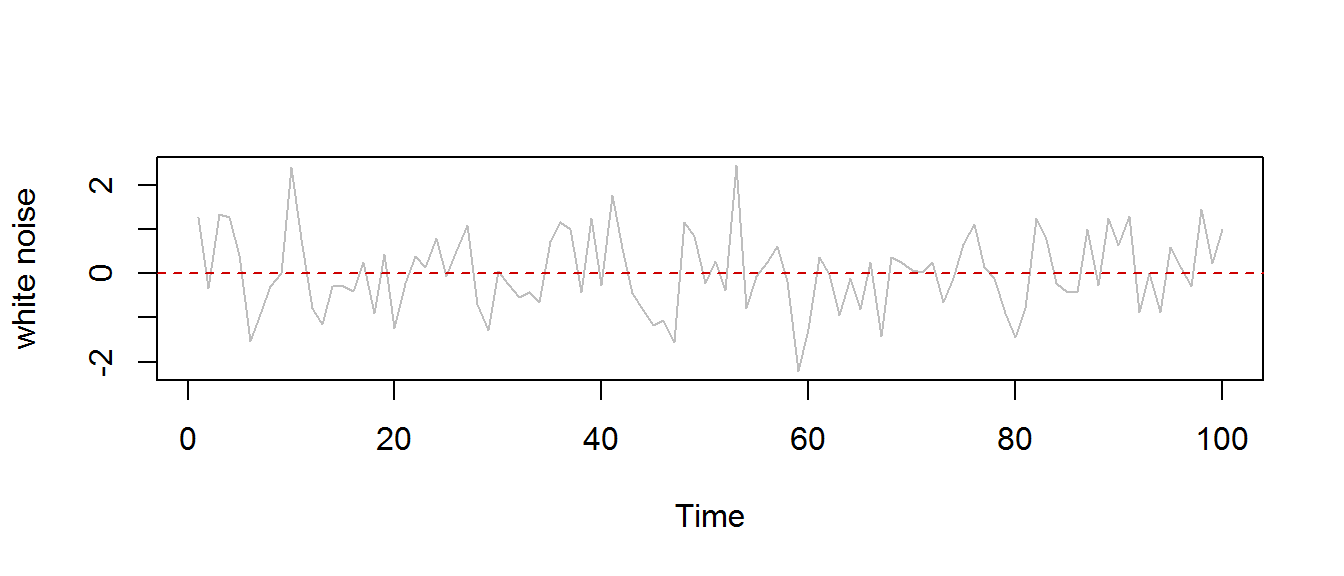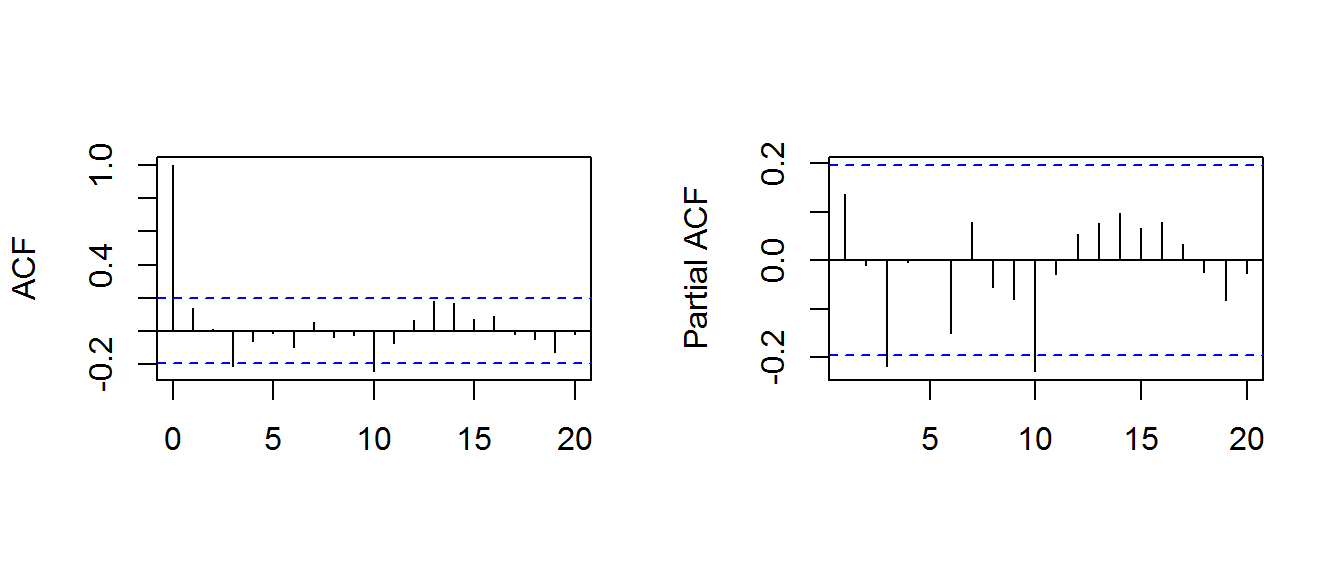#### RANDOM WALK:

A random walk is not stationary because the variance is time-dependent: $$\text{var}[y_t] = \text{var}[y_o]+\sum_1^t \text{var}[\epsilon_i]= t\, \text{var}[\epsilon].$$

$$\large y_t = y_{t-1}+\epsilon_t=\epsilon_t+\epsilon_{t-1}+\cdots+\epsilon_1$$

b = cumsum(rnorm(100))
plot.ts(b, col="chartreuse3", ylab="random walk")
abline(h=0, col="red3", lty=2)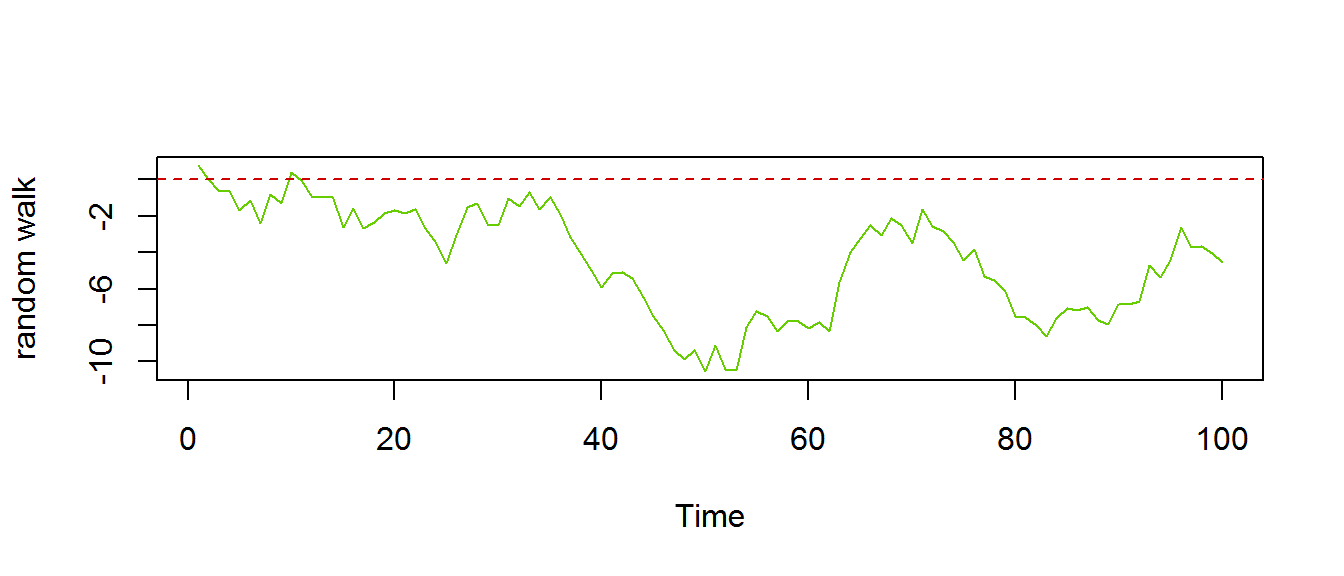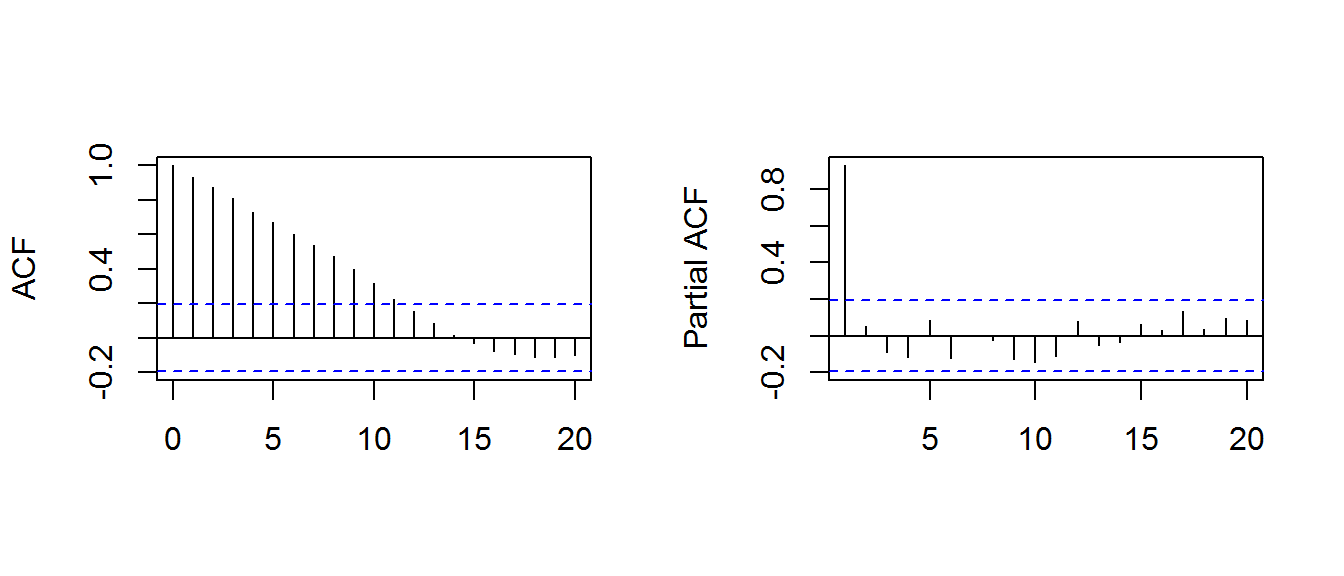To simulate the stock market (and aside from more sophisticted autoregressive and moving average effects), the random walk will not be symmetric (equal probabilities). Here is a random walk during an extremely bullish market:

n=100
x = cumsum(sample(c(1,-1), n, prob = c(0.75, 0.25), replace=T))
plot.ts(x, col="red", ylab="random walk")
abline(h=0, col="red3", lty=2)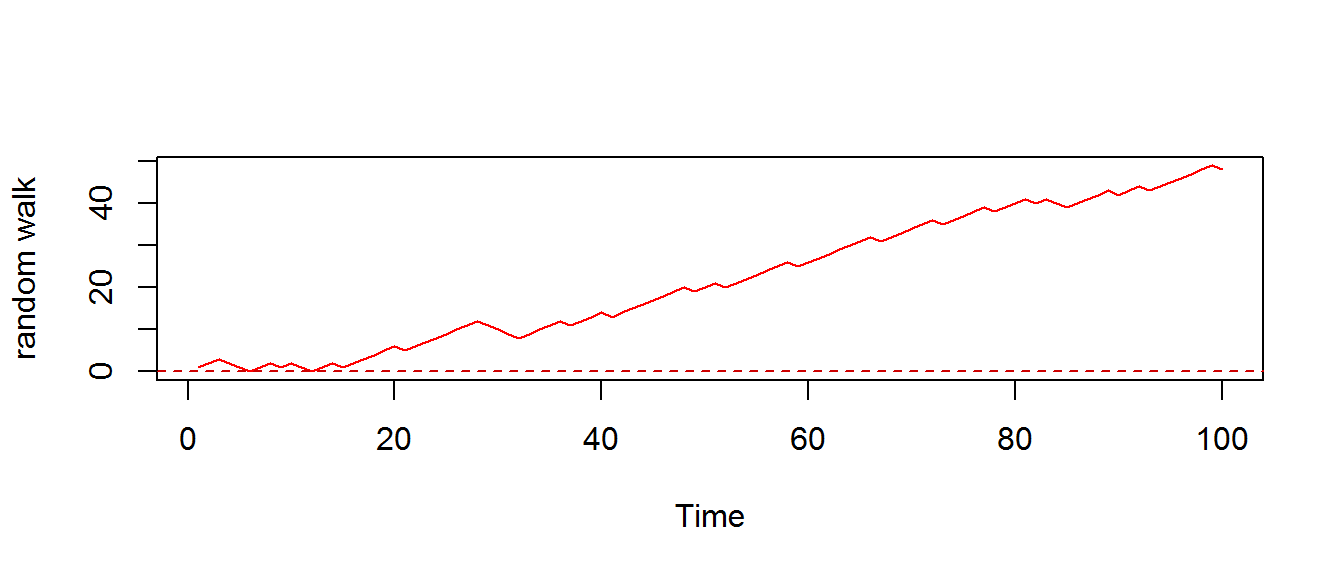#### AUTOREGRESSIVE - AR(1):

$$\large \color{blue}{y_t} = c + \color{red}{\rho}\, \color{blue}{y_{t-1}}+\epsilon_t\tag 1$$

So notice that $$y_t$$ is regressed on itself (this is not the case in MA processes). We can recur the equation:

$$\large \color{blue}{y_{t-1}} = c + \color{red}{\rho}\, \color{blue}{y_{t-2}}+\epsilon_{t-1}\tag 2$$

Substituting 2 into 1:

$$\large \color{blue}{y_t} = c + \color{red}{\rho}\, \left ( c + \color{red}{\rho}\, \color{blue}{y_{t-2}}+\epsilon_{t-1} \right)+\epsilon_t= c + \color{red}{\rho}\, c + \color{red}{\rho^2}\, \color{blue}{y_{t-2}} +\color{red}{\rho} \epsilon_{t-1} +\epsilon_t$$

This “compound interest” expression is not possible in MA.

When $$\rho < 1$$ the series is “covariance stationary”. Notice how the influence of the immediate prior position will be, to a certain extent, countered by the effect of $$\rho$$: the larger $$\rho$$ is the closer AR will be to a random walk; and the smaller it is, the more similar to white noise. $$\epsilon_t$$ can pull in any direction, but a $$\rho < 1$$ pulls back $$y_{t-1}$$ back towards $$0$$.

After turning a time series into an AR series, we’ll be dealing with a stationary dataset, and we can fit a model by selecting optimal parameters, such as $$\rho$$.

Leaving the constant $$c=0$$, and set $$\rho=0.6$$, here is an example:

c = arima.sim(n=100,list(ar=0.6),innov=rnorm(100))
plot.ts(c, col="chocolate", ylab="AR(1)")
abline(h=0, col="red3", lty=2)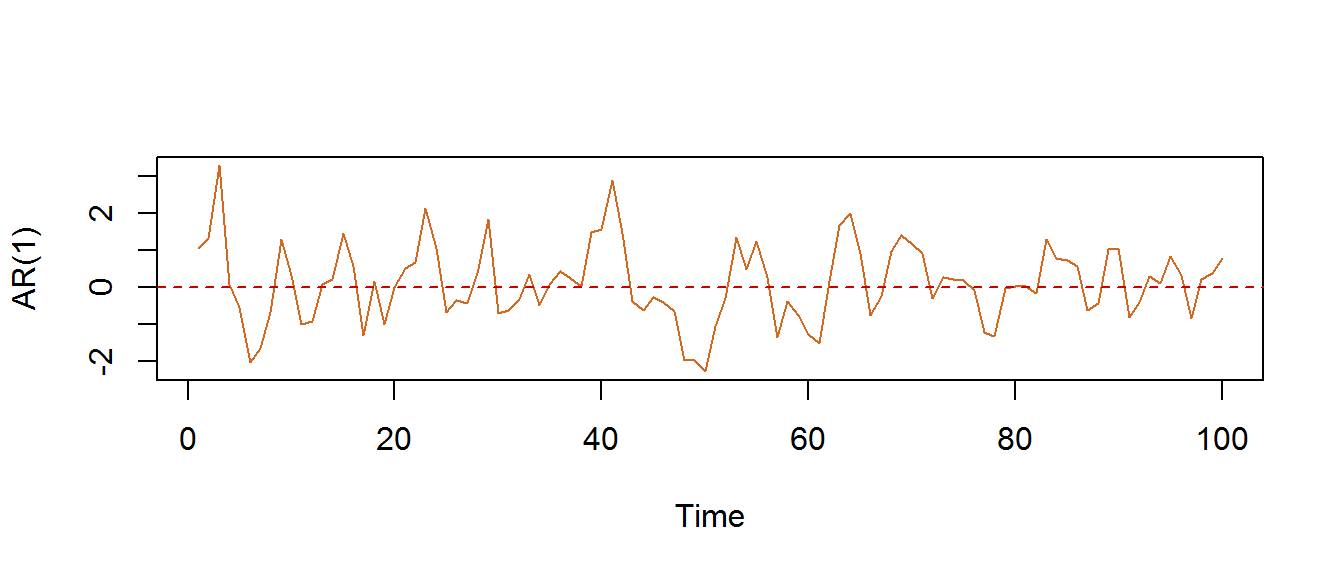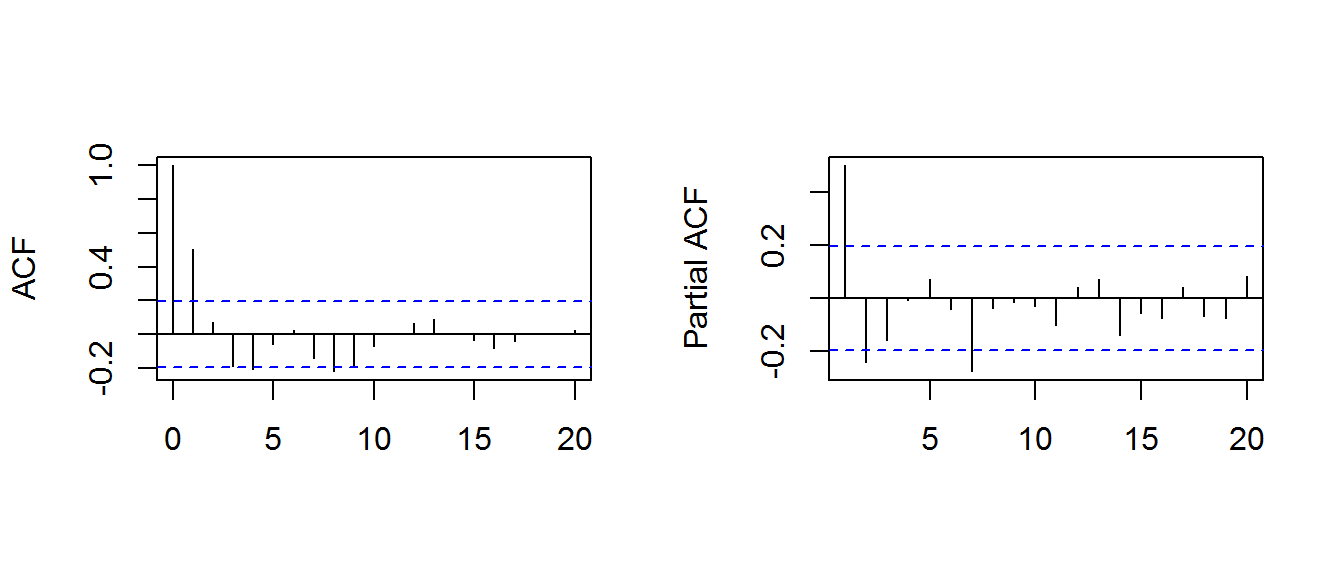Notice how the lag 1 ($$y_{t-1}$$) value is significant. But if we suppress this dependency, there is no further correlation upstream (except for some statistical random outliers). Also, the acf starts off comparing $$t$$ to $$t$$, whereas the first line on the pacf plot already compares $$t$$ to $$t-1$$.

For any $$\text{AR(n)}$$:

$$\large y_t = \color{red}{\rho_1}\, y_{t-1}+ \color{blue}{\rho_2}\, y_{t-2} +\cdots+ \color{gray}{\rho_n}\, y_{t-n}+ c+\epsilon_t$$

In AR(2) we have two lags:

d = arima.sim(n=100,list(ar=c(0.6,-.5)),innov=rnorm(100))
plot.ts(d, col="chocolate", ylab="AR(2)")
abline(h=0, col="red3", lty=2)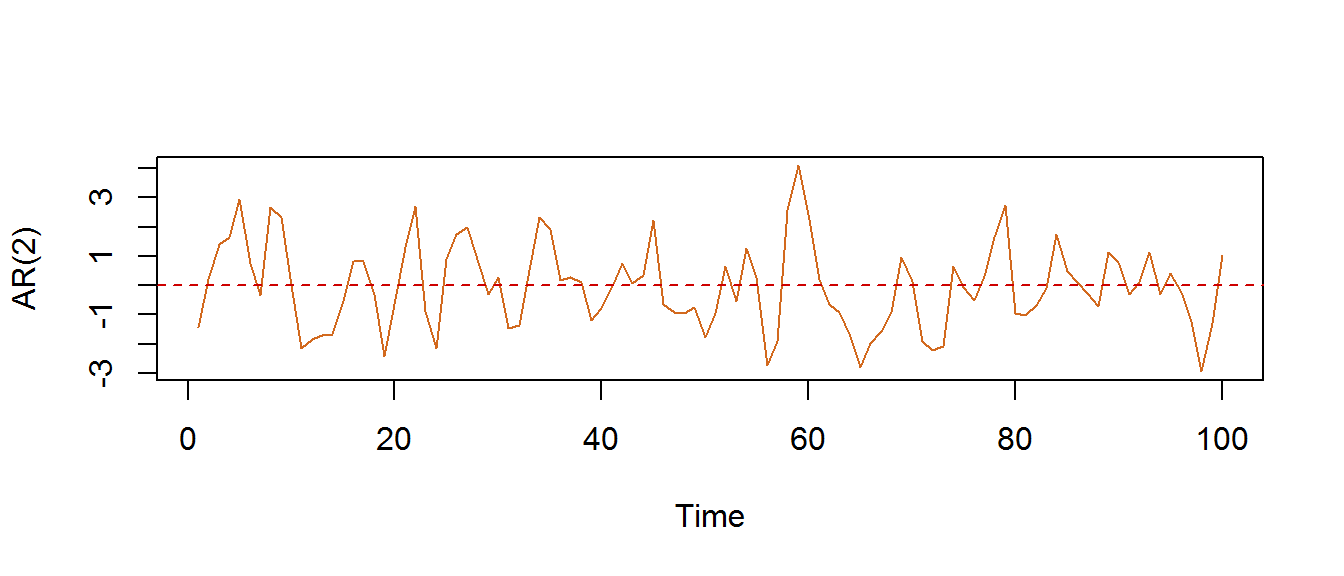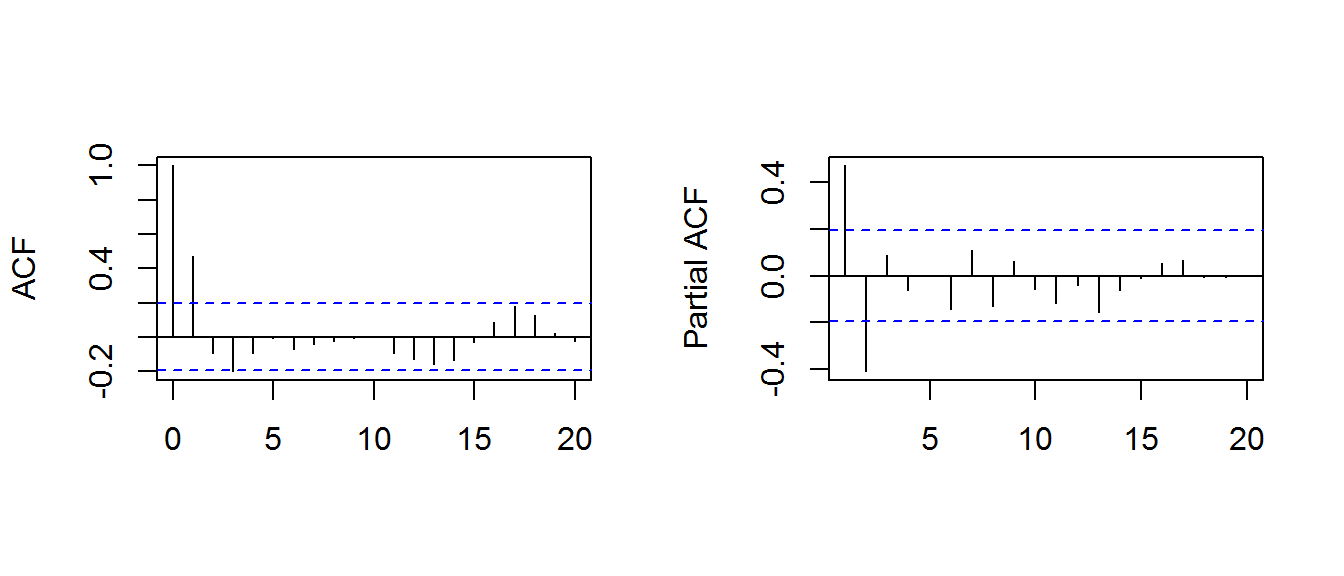Notice the negative second lag related to the $$-0.5$$ AR value. Notice how the acf just gives us a significant correlation without the sign.

#### MOVING AVERAGE or MA:

MA is also stationary, and by affecting noise, it’s effects are less long-lived than AR.

An AR(1) process is defined as:

$$\large\color{blue} {y_t} = k+ \color{red}{\theta}\,\epsilon_{t-1}+\epsilon_t$$

which can clearly be extended to $$MA(n)$$.

Here is an MA(2) process:

e = arima.sim(n=100,list(ma=c(.88,-.48)),innov=rnorm(100))
plot.ts(e, col="cyan", ylab="MA(2)")
abline(h=0, col="red3", lty=2)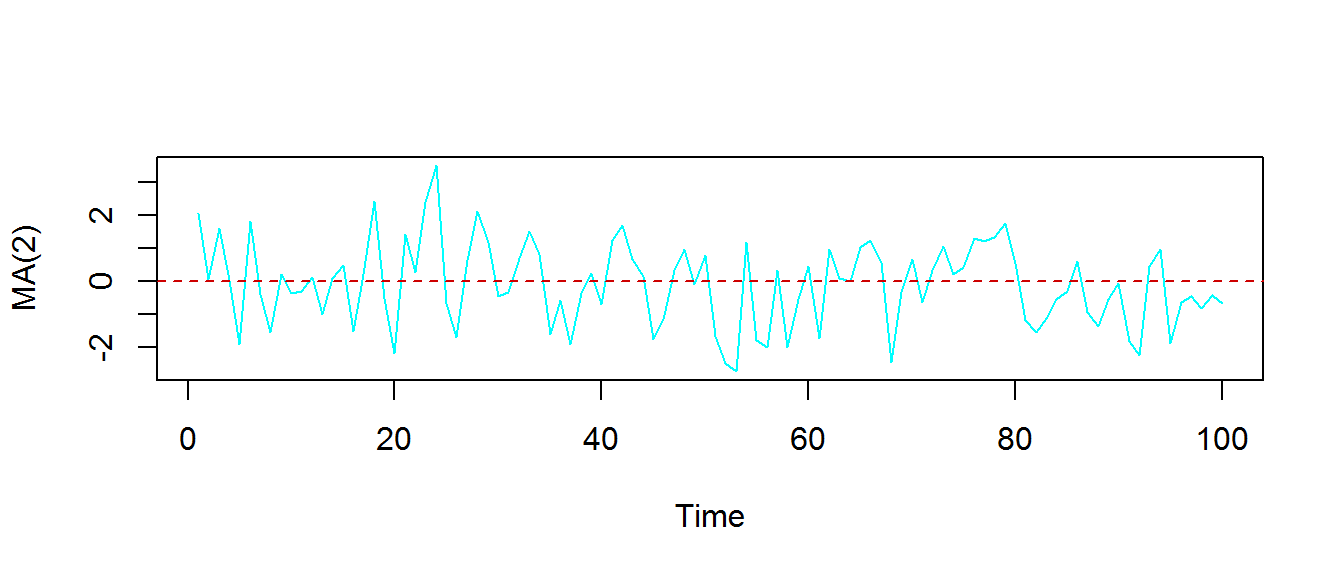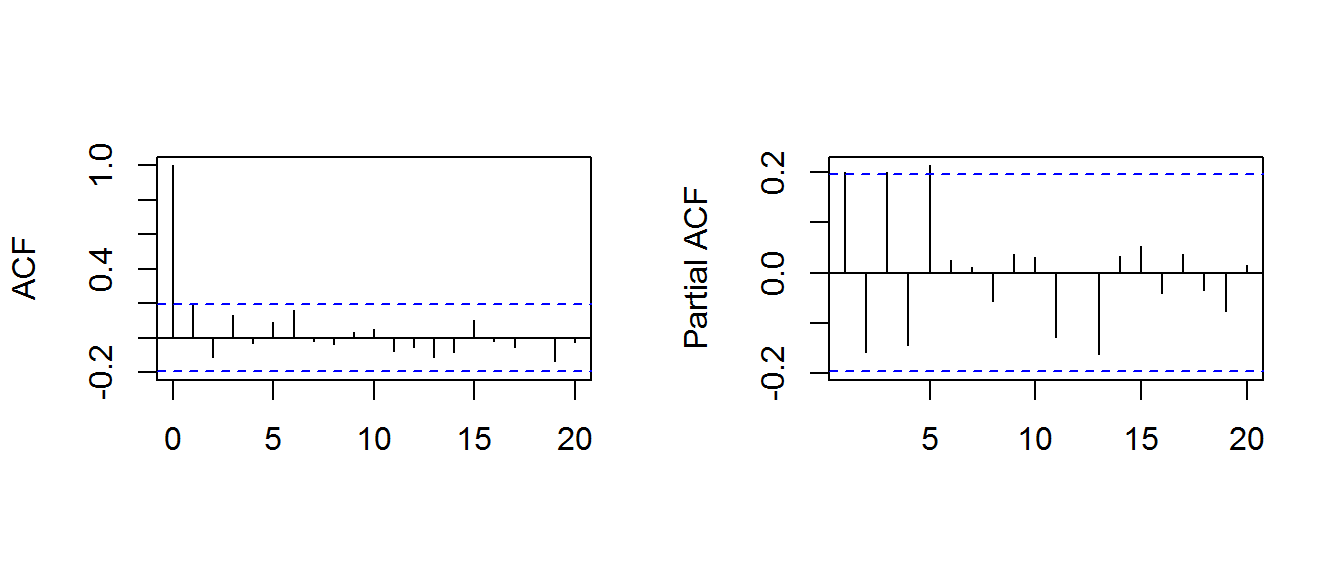#### MIXED MODELS or ARMA Models:

$$\large y_t = C + \color{red}{\rho_1}\, y_{t-1}+ \color{blue}{\rho_2}\, y_{t-2} +\cdots+ \color{gray}{\rho_n}\, y_{t-n}+ \epsilon_t + \color{red}{\theta_1}\, \epsilon_{t-1}+ \color{blue}{\theta_2}\, \epsilon_{t-2} +\cdots+ \color{gray}{\theta_m}\, y_{t-m}$$

Example of ARIMA(2,2):

f = arima.sim(n=100,list(ar=c(0.6,-.5), ma=c(.88,-.48)),innov=rnorm(100))
plot.ts(f, col="blue3", ylab="MA(2)")
abline(h=0, col="red3", lty=2)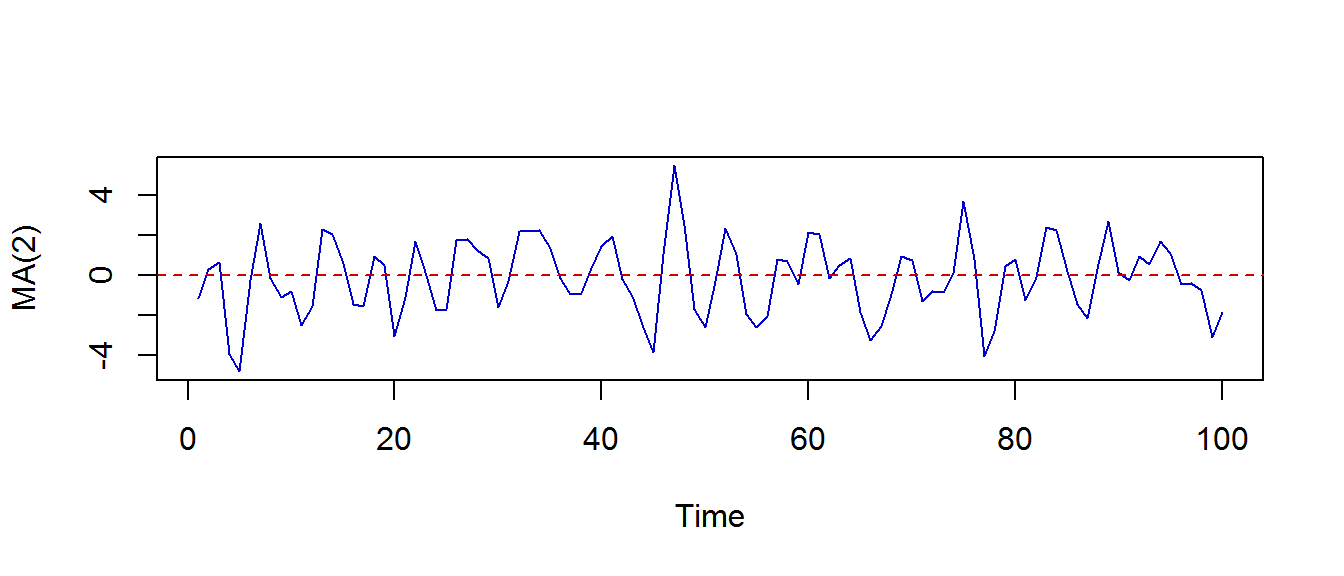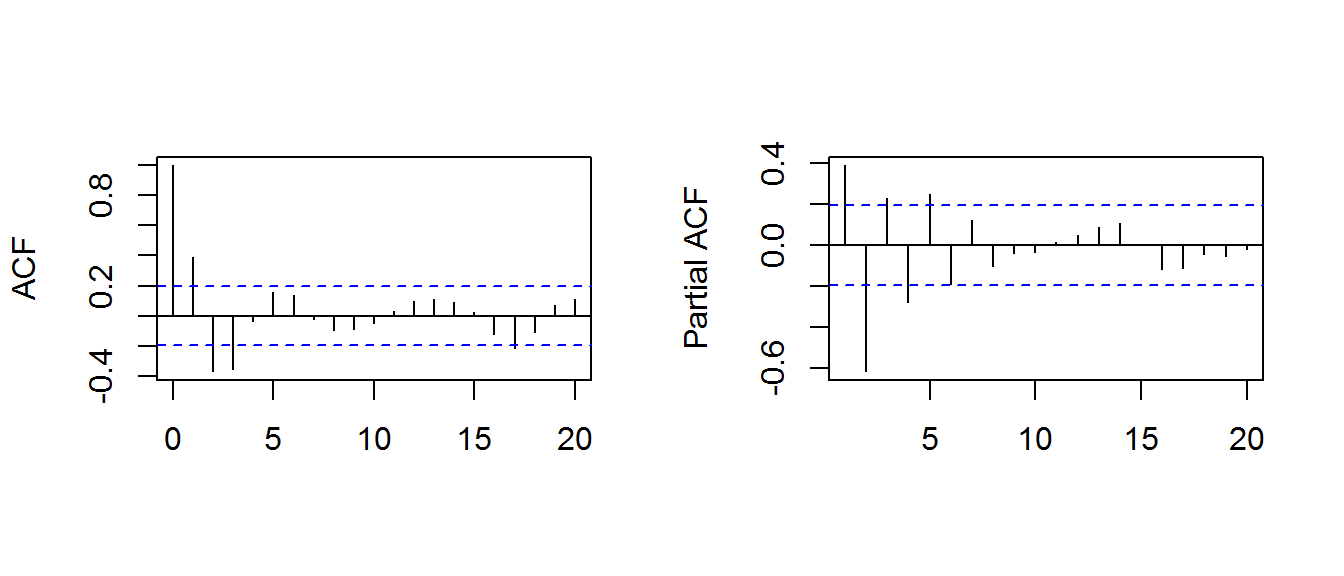In one single shot: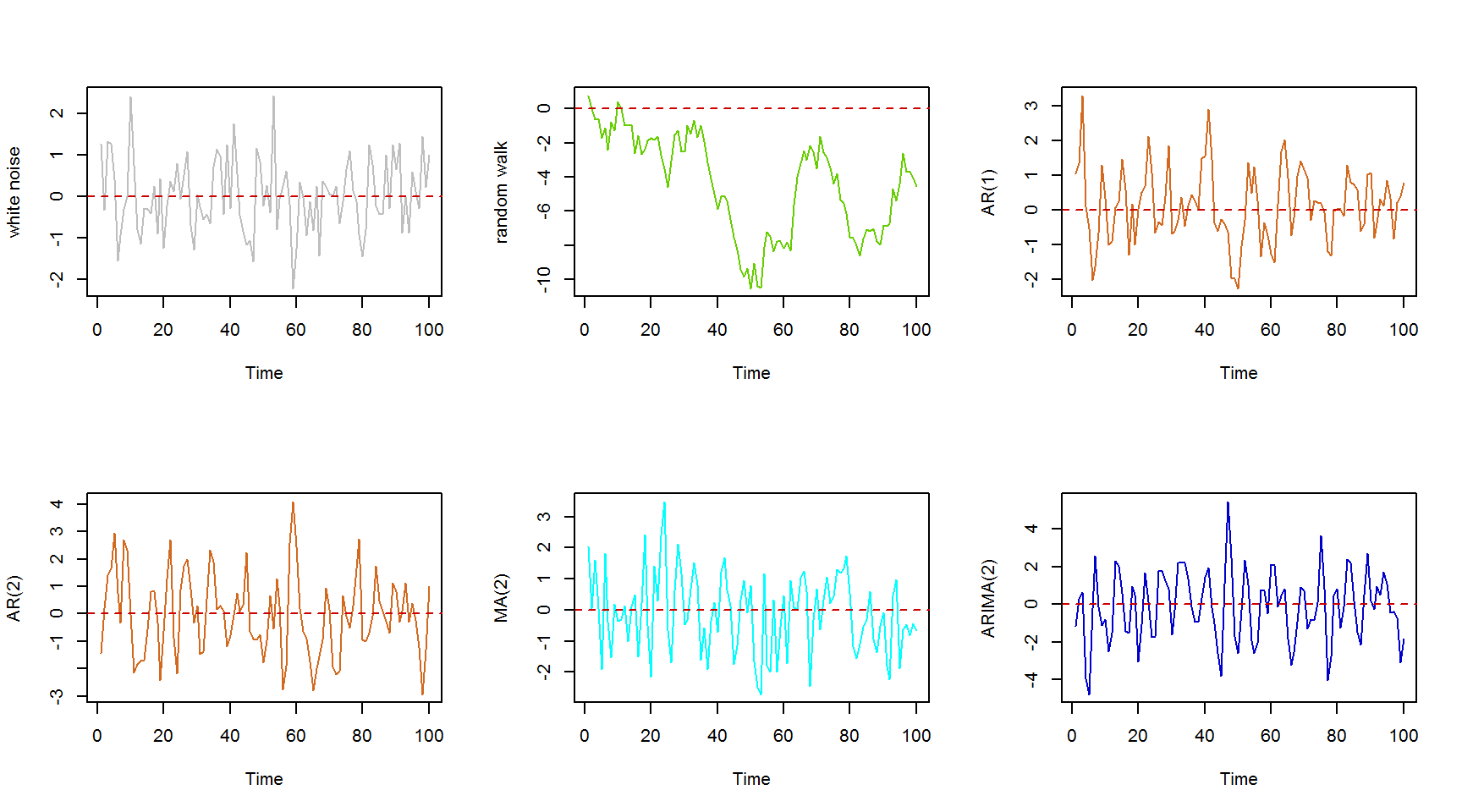So, after having a stationary dataset, the two questions to answer before fitting a model are:

1. Is it an AR or MA process?
2. What order of AR or MA process do we need to use?

In a moving average (MA) series of lag n, we will not get any correlation between $$x(t)$$ and $$x(t – n -1)$$. Hence, the total correlation chart (ACF) cuts off at $$n$$-th lag. So it becomes simple to find the lag for a MA series. For an AR series this correlation will gradually go down without any cut off value.

In an autoregression series (AR), the PACF will cut off after the degree of AR series: if we have a AR(1) series, if we exclude the effect of 1st lag ($$y_{t-1}$$) ), our second lag ($$y_{t-2}$$) ) is independent of $$y_t$$. Hence, the partial correlation function (PACF) will drop sharply after the 1st lag.

The series can be made stationary with one of the following techniques:

1. Detrending
2. Differencing
3. Incorporating seasonality

#### Detrending:

$$y_t = (\text{mean} + \text{trend} \times t) + \text{error}$$

gets rid of the parenthesis part.

#### Differencing:

We model the differences between time points:

$$y_t – y_{t-1} = \text{ARMA (p,q)}$$

Differencing is called as the Integration part in AR(I)MA. Now, we have three parameters p : AR; d : I; q : MA.

#### Seasonality:

Seasonality can easily be incorporated in the ARIMA model directly.

In the case of AirPassengers:

plot.ts(AirPassengers)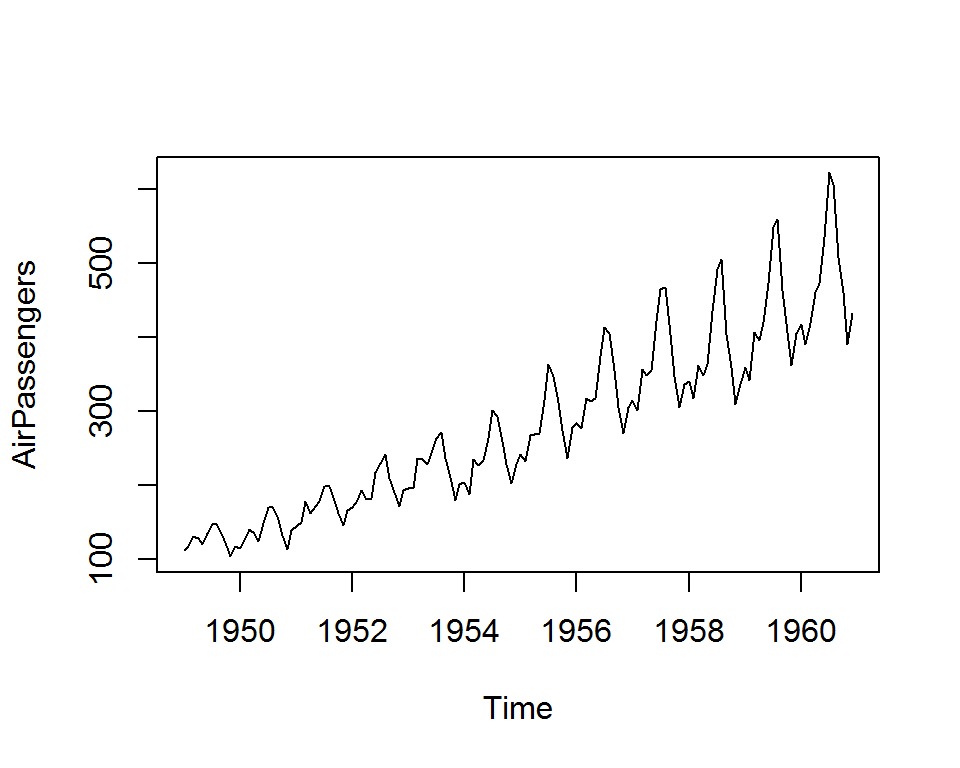we a trend (solution: taking differences), seasonality and increasing variance (solution: $$log$$).

Testing for stationarity now:

## Loading required package: tseries
adf.test(diff(log(AirPassengers)), alternative="stationary", k=0)
##
##  Augmented Dickey-Fuller Test
##
## data:  diff(log(AirPassengers))
## Dickey-Fuller = -9.6003, Lag order = 0, p-value = 0.01
## alternative hypothesis: stationary

Now the data is stationary. But we still need to find the parameters:

acf(log(AirPassengers))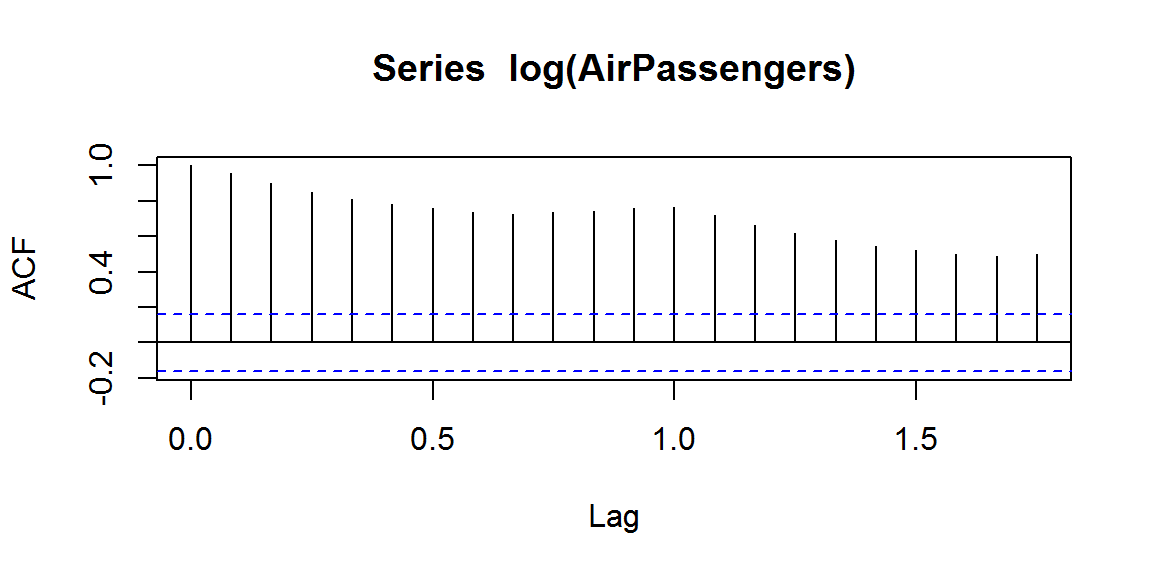Clearly, the decay of ACF chart on the left is very slow, which means that the population is not stationary.

par(mfrow = c(1,2))
acf(diff(log(AirPassengers)))
pacf(diff(log(AirPassengers)))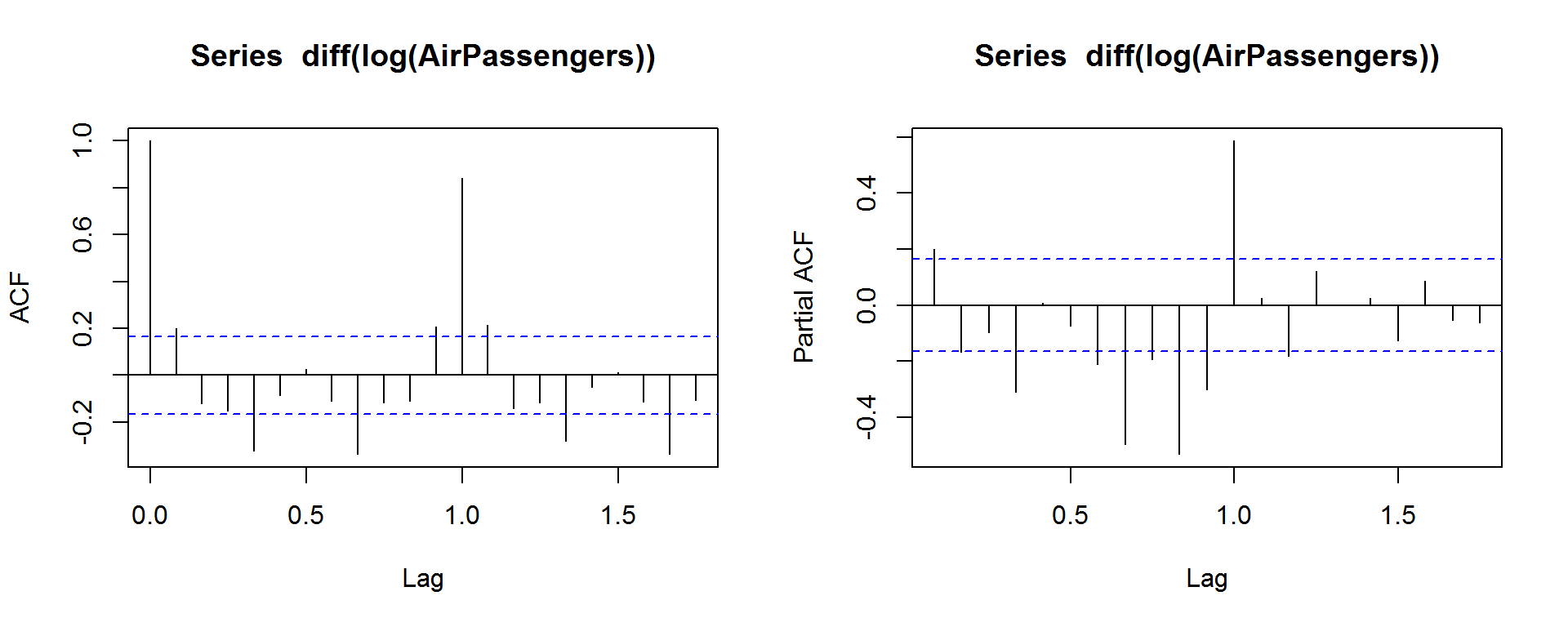The PACF shows many significant values, and there is one significant step in the ACF. Hence we can try fitting a model with a AR parameter of $$0$$ and a MA parameter of $$1$$, i.e. with parameters p, d and q, $$(p,d,q) = (0,1,1)$$:

fit = arima(log(AirPassengers), c(0, 1, 1), seasonal = list(order = c(0, 1, 1), period = 12))

Now we can predict ten years ahead and plot:

pred <- predict(fit, n.ahead = 10*12)
ts.plot(AirPassengers,exp(pred\$pred), log = "y", lty = c(1,3))